# The extra lines: How do you know where to put the extra line that helps you with the question?

• Module 2 Week 2 Day 6 Challenge Part 4 Explanation

How do you know where to put the extra line that helps you with the question?

• @mirthfulostrich said in The extra lines:

How do you know where to put the extra line that helps you with the question?

Thank you for asking!I'm wondering which line you are talking about. Here are some screenshots from the Day 6 Challenge explanation videos. Could you please let me know? Thank you so much!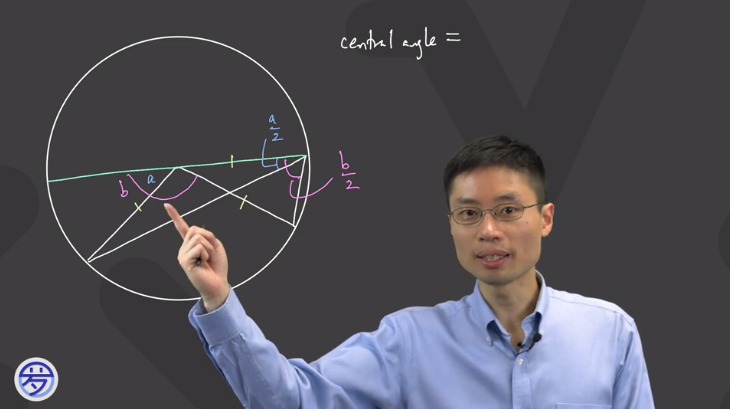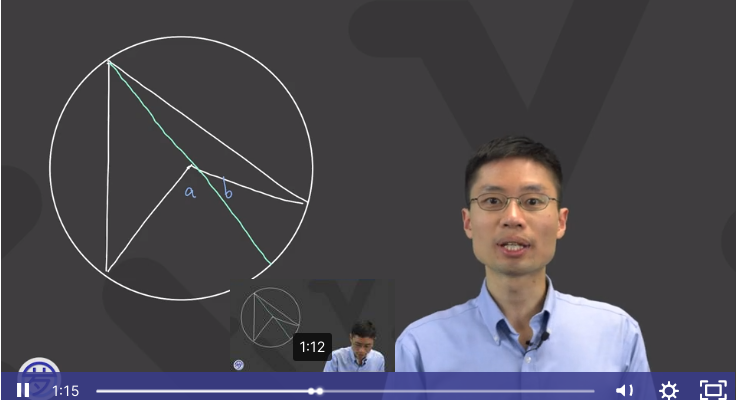• The one on the top.

• @mirthfulostrich Hi, thanks for your reply! The reason Prof. Loh made that $$\textcolor{green}{\text{green}}$$ line is because it's a diameter. Why is that nice? A diameter goes through the center, so this way we get two radii. Why are the radii nice to have? It's because we can then construct isosceles triangles which have two radii as their sides. Why is it nice to have isosceles triangles? It's because isosceles triangles have two equal angles, and we're trying to prove something about the angles in this drawing, in particular, the fact that

$$\text{ An inscribed angle is half the measure of the central angle}$$

(If you're interested in this proof, here is a visual proof of the Inscribed Angle Theorem, and here are some examples of inscribed angles.)

Step by step, here is what Prof. Loh did for his proof:

1. ) We want to show that the angle marked with a $$\textcolor{orange}{\text{yellow}}$$ arrow is half of the measure of the central angle (the one formed by the radii meeting at the center).2.) Draw the $$\textcolor{green}{\text{green}}$$ diameter, and label the angle formed by the $$\textcolor{green}{\text{green}}$$ line and the left-hand radius $$\angle a.$$ The triangle with two radii (marked by the yellow ticks) as sides is isosceles, so the $$\textcolor{purple}{\text{purple}}$$ angles are the same size.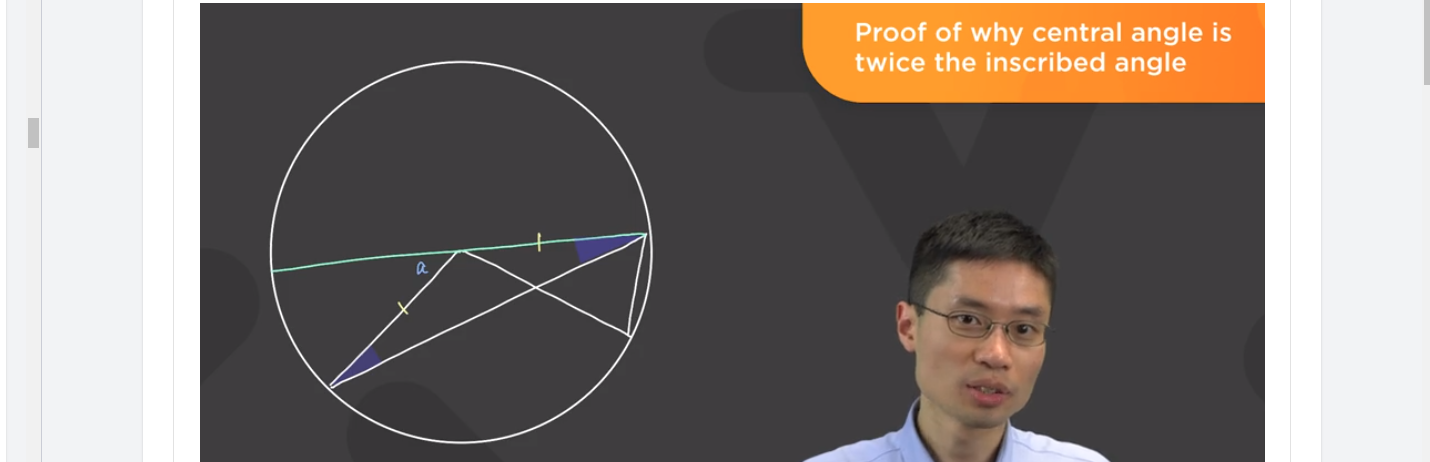3.) Using the Exterior Angle Theorem, we know the $$\textcolor{purple}{\text{purple}}$$ angles add up to $$\angle a,$$ so each of them is equal to $$\angle \frac{1}{2}a.$$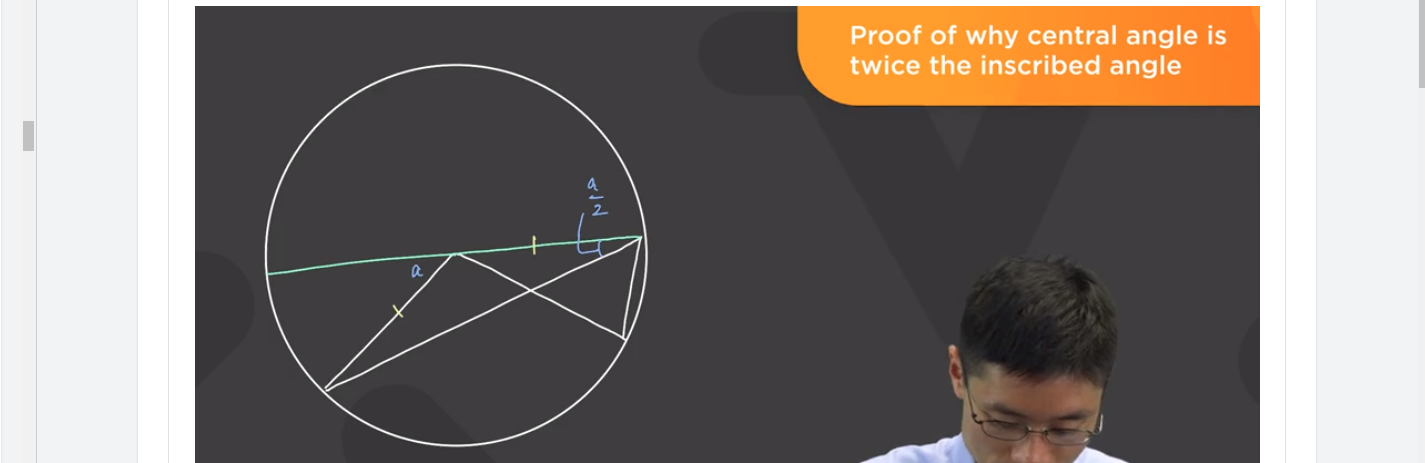4.) Remember, we actually want the angle that was marked $$\textcolor{orange}{\text{yellow}}$$ above, so, in order to get that, we need to label some other angles. What other information can we use in the diagram? Well, there is another isosceles triangle in the picture! It's the one with two other radii as sides, outlined in $$\textcolor{red}{\text{red}}$$ below: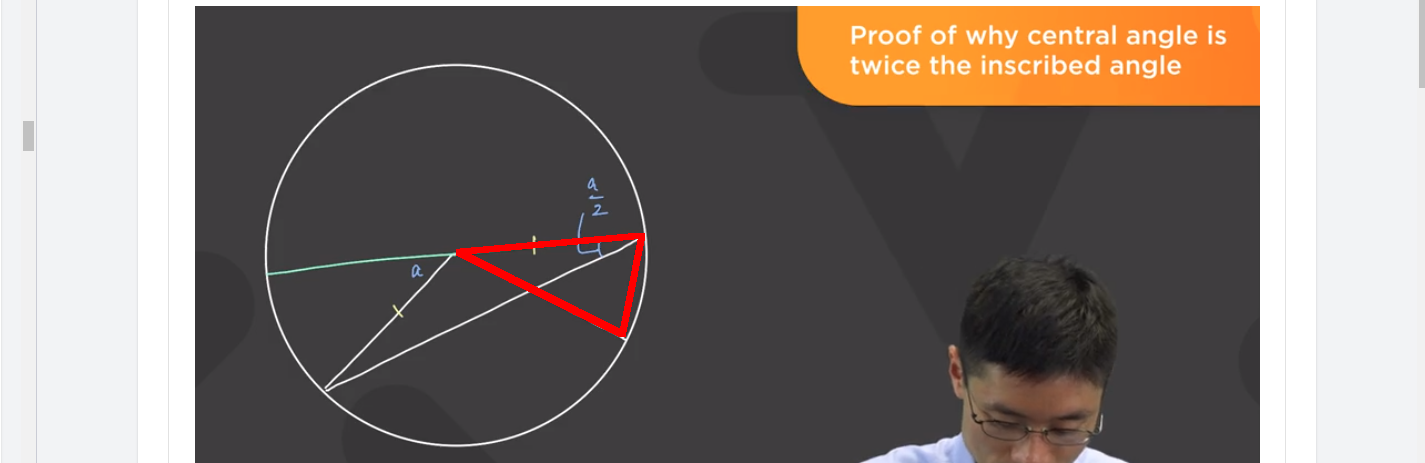5.) One of the larger angles of this triangle is the angle that we want, but first, we need to find some other angle that relates to this triangle. That's why Prof. Loh made the angle $$\angle b,$$ labeled in $$\textcolor{pink}{\text{pink}}.$$ This (\textcolor{pink}{\text{pink}}\) angle is an exterior angle of the $$\textcolor{red}{\text{red}}$$ triangle. Using the Exterior Angle Theorem again, each of the larger angles of this triangle is equal to half of the exterior angle, so each is $$\angle \frac{1}{2} b.$$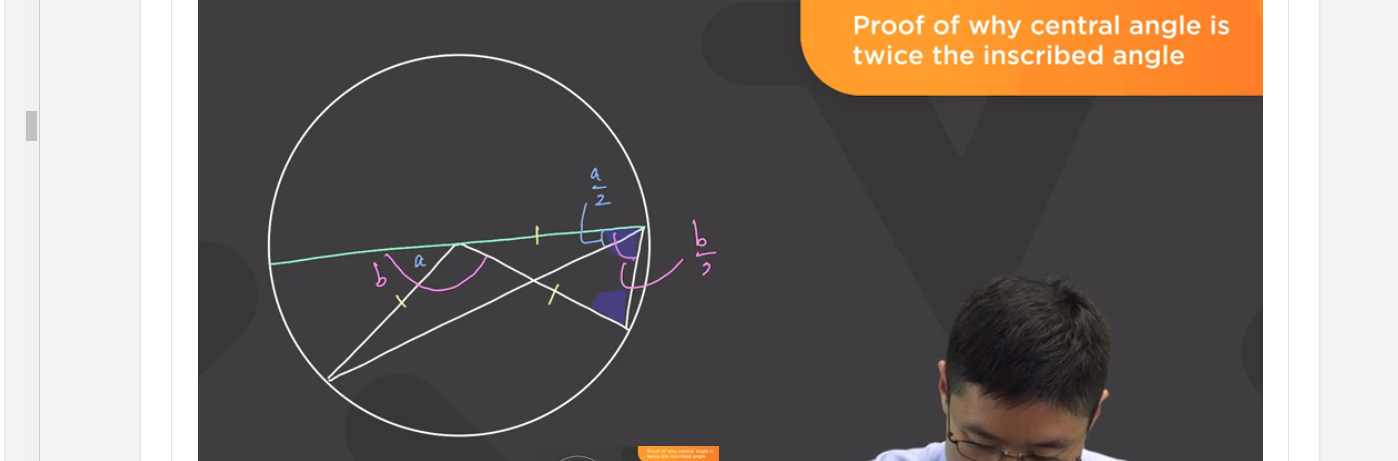6.) The end is in sight! The angle that we want is the $$\textcolor{pink}{\text{pink}}$$ angle, $$\angle \frac{1}{2} b,$$ minus the $$\angle \frac{1}{2} a$$ angle, or

$$\text{ Angle we want } = \frac{1}{2} (b-a)$$

7.) What were we trying to prove again? We were trying to show that an inscribed angle is half of the central angle. (Remember that these angles are inscribing the same arc of the circle.) In the case of our diagram, we were trying to show that the $$\textcolor{orange}{\text{yellow}}$$ angle is half of the $$\textcolor{green}{\text{green}}$$ angle.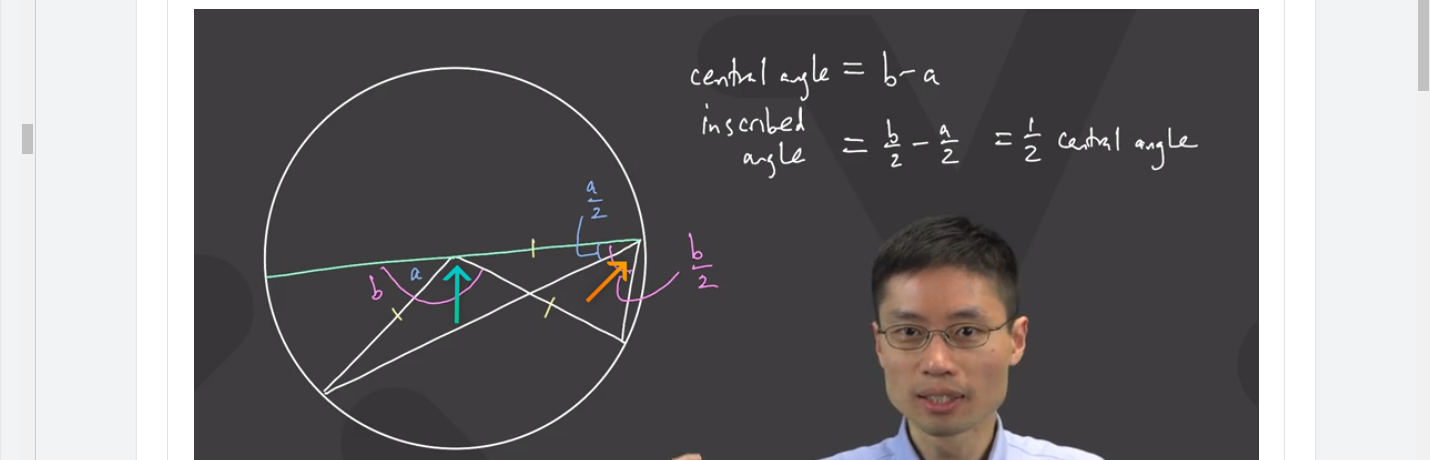Well, what is the $$\textcolor{green}{\text{green}}$$ angle? It is equal to $$\angle b - \angle a.$$
And since the $$\textcolor{orange}{\text{yellow}}$$ angle is $$\frac{1}{2} (b-a),$$ it is indeed true that the $$\textcolor{orange}{\text{yellow}}$$ angle is half of the $$\textcolor{green}{\text{green}}$$ angle. Hooray, so does this prove the Inscribed Angle Theorem? Well, yes! Even if you draw the lines at different angles, these relationships still hold.